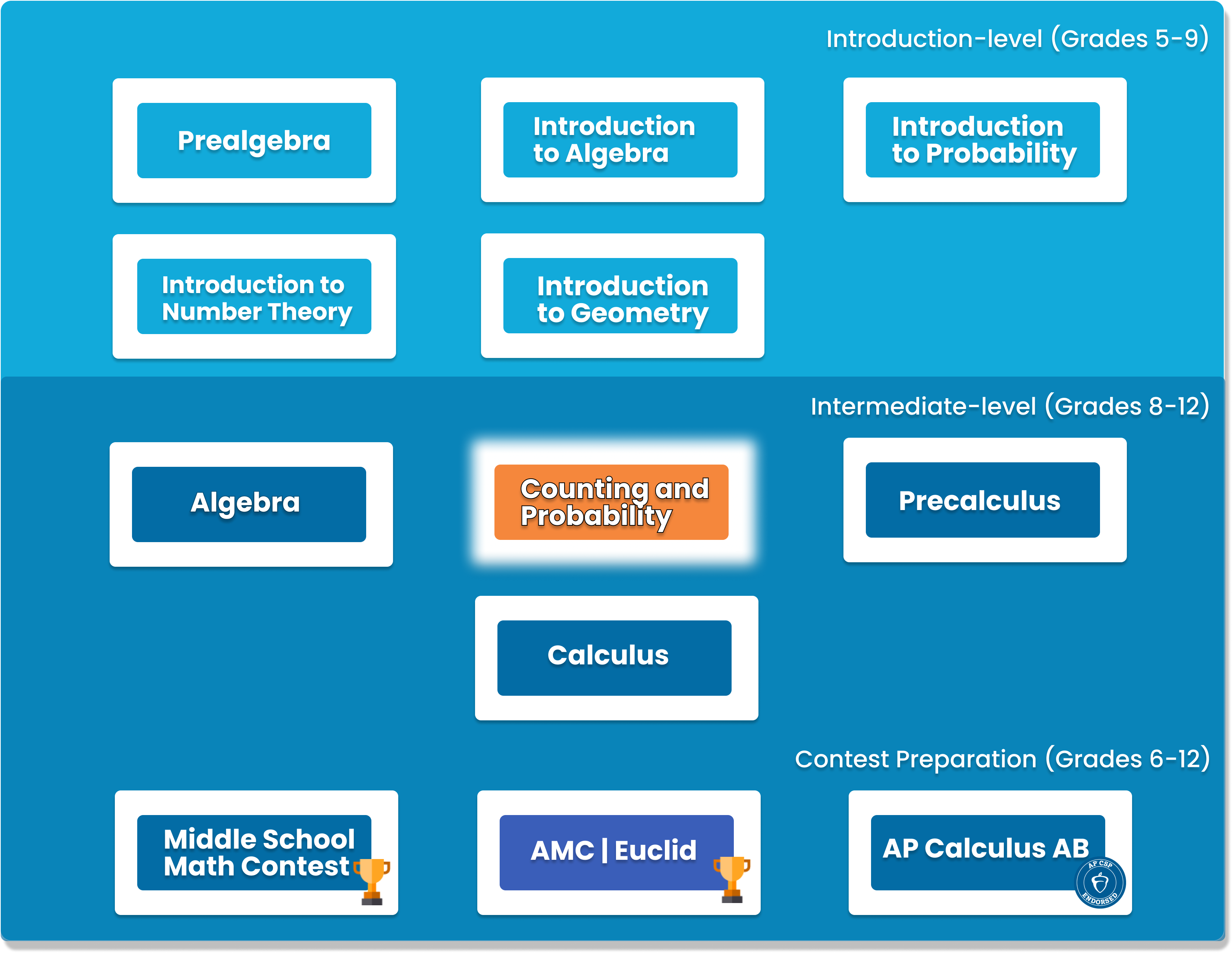# Counting and Probability

To build on the concepts from the Introduction level course, students will build on their Counting and Probability knowledge. A thorough introduction for students in grades 7-10 to counting and probability topics such as permutations, combinations, Pascal's triangle, geometric probability, basic combinatorial identities, the Binomial Theorem, and more. Pigeonhole Principle Conditional Probability Graph Theory##### Who is this course for
This course is for students who have completed our Introduction to Counting and Probability course.
This course typically takes 3-6 months to complete. This depends on the student's experience and how fast they can master the concepts and the knowledge. Our instructors move at the pace of the student, it may take extra time for some students to reinforce what they have learned.
##### Curriculum
###### Chapter 1: Review of Counting and Probability BasicsReview of basic counting and probability, expected value, Pascal’s Triangle and Binomial Theorem, and the summation notation
###### Chapter 2: Sets and LogicSets, logics, quantifiers
###### Chapter 3: A Piece of PIEPrinciple of Inclusion and Exclusion
###### Chapter 4: Constructive Counting and 1-1 correspondancesConstructive counting, count by finding1-1 correspondances
###### Chapter 5: Pigeonhole PrinciplePigeonhole Principle
###### Chapter 6: Constructive ExpectationLinearity of Expectation
###### Chapter 7: DistributionsCount the number of ways to place indistinguishable items into distinguishable boxes
###### Chapter 8: Mathematical InductionInduction
###### Chapter 9: Fibonacci NumberIntroduction and application for Fibonacci Numbers
###### Chapter 10: RecursionRecursion, Catalan Numbers
###### Chapter 11: Conditional ProbabilityConditional Probability, and their applications
###### Chapter 12: Combinatorial IdentitiesStrategies of proving Combinatorial Identities
###### Chapter 13: Events with statesState diagrams, random walks, events with infinite states
###### Chapter 14: Generating FunctionsDefinition, and applications in deriving the binomial theorem, as well as formulas for distribution, partitions, and Fibonacci numbers
###### Chapter 15: Graph TheoryDefinitions, Basic Properties, Cycles and Paths, Planar Graphs, Eulerian and Hamiltonian paths
###### Chapter 16: Challenge ProblemsHard problems in counting that use combinations of topics above

### You Might Also Be Interested In Our Elective Courses##### Python Level 1
AGES 10 - 15

Designed for beginners, this level is designed to teach the basic fundamentals and design principles of Python, with the help of Turtle graphics and PyGame. This includes: variables, conditional statements, loop basics, and functions.

These concepts are transferable to any other programming language. Throughout the way, students will create projects in order to apply the concepts they have learned, and to solidify their knowledge.##### Python Level 2
AGES 10 - 15

Level 2 dives deeper into the basics of Python for a more thorough understanding and introduces advanced topics. Students will build on knowledge from Level 1 and work with data structures, advanced loops, algorithms, and object-oriented programming, and create games based on what they learn.

Students will complete the course with a solid understanding of Python fundamentals.##### Java Level 1
AGES 10 - 15

This beginner-friendly course serves as an introduction to the Java programming language. Students will learn the fundamentals of Java along with core computer science concepts. Concepts covered in this course include Java syntax, types and identifiers, operators, if statements, loops, arrays and more.

Students will apply these concepts to create fun games such as Tic-Tac-Toe.